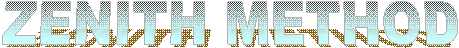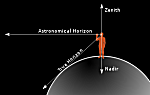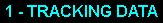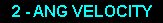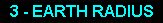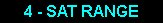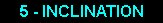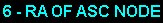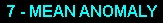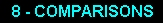The "Zenith Method" of Low Earth Orbit (LEO) satellite initial orbit determination was originally derived by the author when it was necessary to determine the range of LEO satellites using endpoint observations of a single streak in an image obtained when the satellite appeared at or near the local zenith. This method is especially useful for those satellites that do not have published orbit elements and subsequent observations are required for more accurate orbit determination. The initial research paper, published in 2006, that described the ranging of a LEO satellite observed at the local zenith, can be found here.

By determining the range of a satellite at or near the observer's local zenith, the semi-major axis of the orbit can also be determined as long as the satellite has a roughly circular orbit (eccentricity of nearly 0). Most low-altitude LEO satellites fit this category because a high-eccentricity LEO orbit would mean a perigee of below the Earth's surface.

This method is much less involved than Gauss' Method and only four of the six Keplerian elements can be found. The eccentricity is assumed to be near zero and therefore the argument of perigee is undefined. Assuming a circular orbit, the eccentricity and argument of perigee are not as important as the orbit radius (or the orbit period), inclination, right ascension of the ascending node (RAAN) and mean anomaly when determining the orbit.

This method might be useful for educators who wish to demonstrate and/or introduce initial orbit determination without the complexity of Gauss' Method, which relies heavily on 8th order equation root solving and matrix/vector algebra.

 CASTOR HOME SITE MAP Initial Orbit Determination - Zenith Method Was Last Modified On December 30, 2013